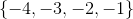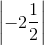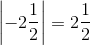# PSAT Math : How to add negative numbers

## Example Questions

### Example Question #1 : Negative Numbers

How many elements of the setare less than?

Two

None

One

Four

Three

Four

Explanation:

The absolute value of a negative number can be calculated by simply removing the negative symbol. Therefore,All four (negative) numbers in the setare less than this positive number.

### Example Question #14 : Negative Numbers

a, b, c are integers.

abc < 0

ab > 0

bc > 0

Which of the following must be true?

a + b < 0

ac < 0

a > 0

b > 0

a – b > 0

a + b < 0

Explanation:

Let's reductively consider what this data tells us.

Consider each group (a,b,c) as a group of signs.

From abc < 0, we know that the following are possible:

(–, +, +), (+, –, +), (+, +, –), (–, –, –)

From ab > 0, we know that we must eliminate (–, +, +) and (+, –, +)

From bc > 0, we know that we must eliminate (+, +, –)

Therefore, any of our answers must hold for (–, –, –)

This eliminates immediately a > 0, b > 0

Likewise, it eliminates a – b > 0 because we do not know the relative sizes of a and b. This could therefore be positive or negative.

Finally, ac is a product of negatives and is therefore positive. Hence ac < 0 does not hold.

We are left with a + b < 0, which is true, for two negatives added must be negative.